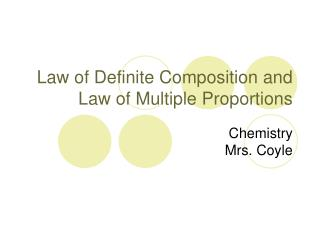DownloadDownload PresentationLaw of Definite Composition and Law of Multiple Proportions

# Law of Definite Composition and Law of Multiple Proportions

Télécharger la présentation## Law of Definite Composition and Law of Multiple Proportions

- - - - - - - - - - - - - - - - - - - - - - - - - - - E N D - - - - - - - - - - - - - - - - - - - - - - - - - - -
##### Presentation Transcript

1. Law of Definite Composition and Law of Multiple Proportions ChemistryMrs. Coyle

2. Joseph Proust (France 1799) • Law of Definite Proportions: A given compound always contains elements in a certain proportion by mass. (Constant composition).

3. Atoms combine in whole number ratios, so their proportion by mass will always be the same. • Example: H2O is always made up of 2 atoms of H and one atom of O. The ratio of O to H in water is always 16:2 or 8:1.

4. Example: • KCl always contains one atom of K for every one atom of Cl • In KCl, potassium and chlorine always have a ratio of “39.09 to 35.45” or “1.1 to 1” by mass.

5. Law of Multiple Proportions (John Dalton) • When the same two elements combine to form more than one compound: the ratios of the mass ofone element in the first compound to its mass in the second compound, (as it combines with the same mass of the other element), can always be expressed as ratios of small whole numbers( ex: 1:3 or 2:5).

6. Example of Law of Multiple Proportions • Carbon combines with oxygen to form CO and CO2 .

7. Practice Problem 1 In the carbon compounds ethane (C2H6) and ethene (C2H4), what is the lowest whole number ratio of H atoms that react with the same number of C atoms? Answer: 3:2

8. Practice Problem 2 Carbon reacts with oxygen to form two compounds as shown: Compound A: 2.41 g C, 3.22 g O. Compound B: 6.71 g C, 17.9 g O. Find the lowest whole number ratio of C that react with an equal mass of O.

9. Solution Strategy • Find the grams of carbon per 1 g of oxygen for each compound. • Divide the highest answer by the smallest answer. Answer: 2:1

10. Practice Problem 3 Lead forms two compounds with oxygen as shown: Compound A: 2.98 g Pb, 0.461 g O. Compound B: 9.89 g Pb, 0.763 g O. For a given mass of oxygen, what is the lowest whole number mass ratio of lead in the two compounds? Answer: 2:1